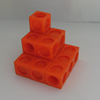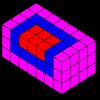#### You may also like### The Root of the Problem

Find the sum of this series of surds.### Sums of Powers - A Festive Story

A story for students about adding powers of integers - with a festive twist.### Summing Squares

Discover a way to sum square numbers by building cuboids from small cubes. Can you picture how the sequence will grow?

# Picture Story

### Why do this problem?

This problem encourages visualisation of a three dimensional idea in a two dimensional context. It offers a visual demonstration of the sum of cubes which can lead to a proof. By extending the picture, ideas of proof by induction could be introduced.

### Possible approach

This printable worksheet may be useful: Picture Story.

Perhaps start by exploring the problem Picturing Triangle Numbers, which develops the formula for the nth triangular number using a pictorial approach.

Ask students to imagine that they are building cubes of different sizes from smaller cubes.

How many small cubes will be required to make a 1 by 1 by 1 cube?
How many small cubes will be required to make a 1 by 1 by 1 cube and a 2 by 2 by 2 cube?
How many small cubes will be required to make a 1 by 1 by 1 cube and a 2 by 2 by 2 cube and a 3 by 3 by 3 cube?
...
Discuss anything that they have noticed, and ask for predictions for constructing a set of all the cubes up to 10 by 10 by 10.

Are they surprised by the patterns they have noticed?

One way of representing this result is using the image provided in the problem. Hand out copies of this worksheet, and ask students to spend some time thinking about how the image relates to the formula. Encourage them to use multilink cubes or draw diagrams to show how the sum of cubes is represented in the image.

Can they draw similar images or extend the existing image to represent the sum of the first 7, 8, 9, 10 cubes? Does this support their predictions above?

Can they now deduce the general formula for the sum of the first n cube numbers? In small groups, they could develop pictures to support a proof of their generalisation, which could be presented to the rest of the class.

### Key questions

Where are the cube numbers in the picture?

How does the picture show $(1+2+3+4+5+6)^2$?
Could you draw similar pictures for other sums of cubes? Can you always draw such a picture?

### Possible support

Try the problems Picturing Triangle Numbers and Picturing Square Numbers.

### Possible extension

The problem Summing Squares offers a similar visual proof idea in three dimensions for the sum of the first n square numbers. Students could read the article Proof by Induction to find out more about this important method of proof.